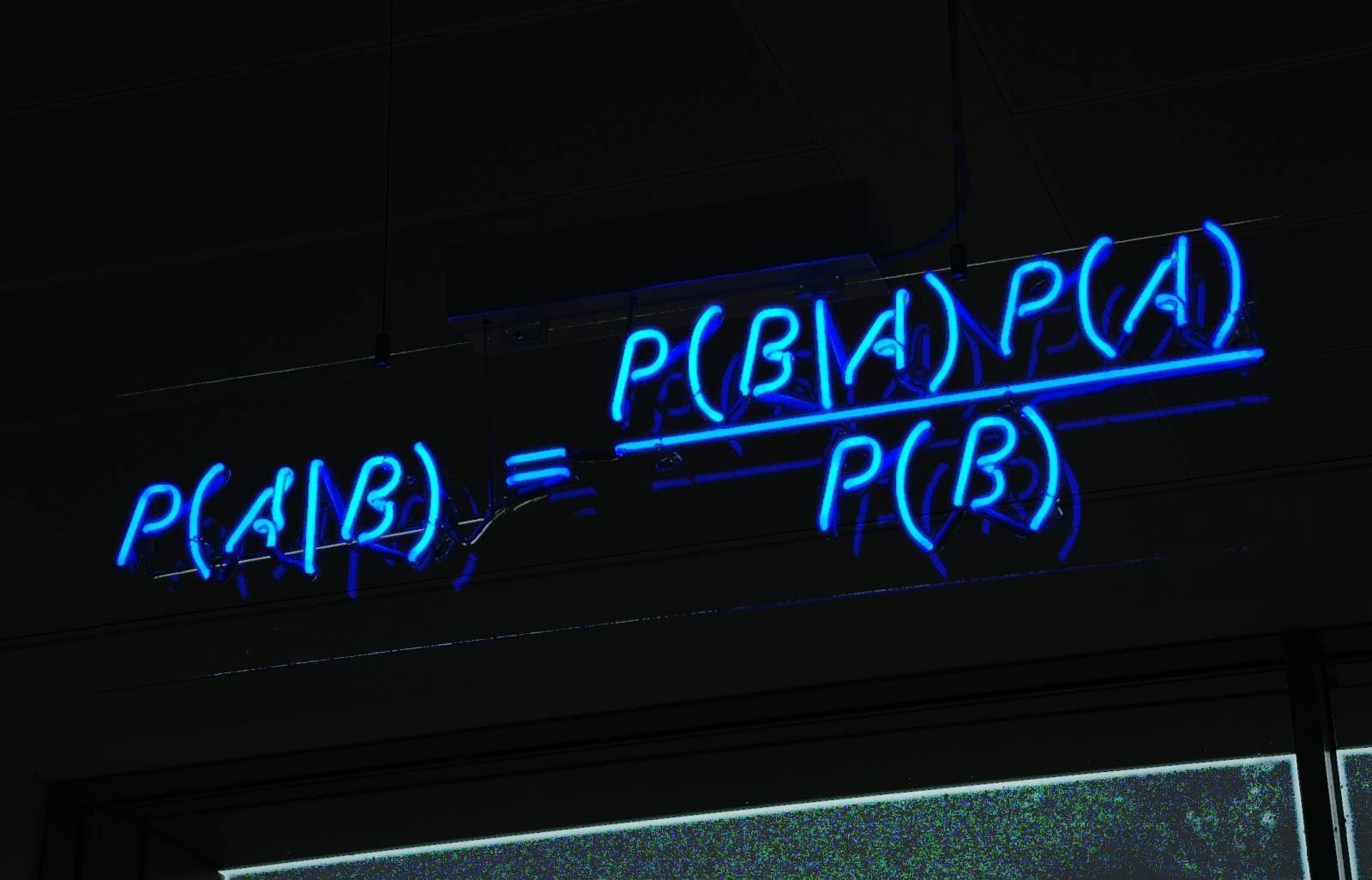# The Mathematics Behind Naive Bayes Classifiers

Original article was published by Taha Binhuraib on Deep Learning on Medium## The Theory Behind The Naive Bayes classifier

The Naive Bayes classifier is a probabilistic classifier that is based on the Bayes’ Theorem with the assumptions that each feature makes an independent and an equal contribution to the outcome. To give an example of the independence assumption, the following analogy would suffice: If we roll a dice n times and we are interested in calculating the probability of getting n 3’s in a row. The probability will be calculated as: (1/6)ⁿ the rolls don’t impact the probability of the subsequent rolls. Regarding the equal contribution assumption, I would like to give an example from natural language processing. Let’s say that you are classifying SMS messages as spam or not spam; each word in our vector of words will constitute a feature. Given equal contribution, each word will have the same “weight” in determining whether an SMS message is to be classified as spam or not spam.

Bayes’ Theorem is mathematically formulated as the following:

In simple terms, our aim is to find the probability that an SMS is spam or not given that it contains a set of words(input data). The open form of the equation above takes the following form:

What we did above is known as the joint probability model; multiplying the probability that word x₁ appears given that the SMS is spam with the word x₂ and so on.. This, of course, is possible thanks to the independence assumption we made earlier. When calculating the probability, the numerator is of little importance, since we could regard it as a constant as it does not depend on y.

The classifier model can be expressed as the following:

Here we are simply looking for the class {0,1} that will maximize the probability of that class multiplied by the probabilities of the feature vectors given that that class.

In the upcoming article we will take a real life example from NLP and apply Naive Bayes using the scikit-learn library